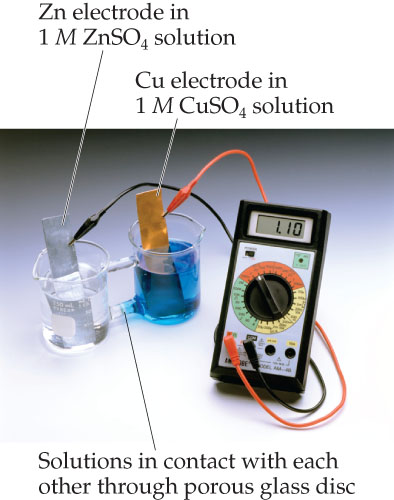# Problem: A Cu-Zn voltaic cell based on the reaction in the equation given below.Zn( s ) + Cu2 +  ( aq )  →  Zn2 +  ( aq ) + Cu( s )Which metal is oxidized in this voltaic cell?

###### FREE Expert Solution

We’re being asked to determine which metal is being oxidized given the following reaction.

Zn(s) + Cu2+(aq) → Zn2+(aq) + Cu(s)

Recall the mnemonics LEO GER.

Lose               Gain

Electron         Electrons

Oxidation       Reduction

We’ll identify the anode and cathode half-reactions using the following steps.

Step 1. Separate the overall reaction into two half-reactions
Step 2. Balance the elements in each half-reaction.

79% (327 ratings)###### Problem DetailsA Cu-Zn voltaic cell based on the reaction in the equation given below.
Zn( s ) + Cu2 +  ( aq )  →  Zn2 +  ( aq ) + Cu( s )

Which metal is oxidized in this voltaic cell?